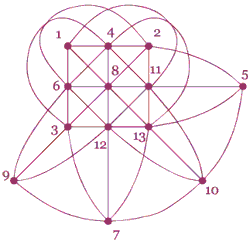High School Mathematics ContestContest Home > Contest Logo -> 2004 Contest Logo

## 2004 Contest LogoWe owe the drawing of the T-shirt design and the 2004 contest logo to professor James Oxley from LSU Math Department. It portrays a finite projective plane of order 3 which consists of 13 points.

Projective Geometry is a geometry that is different from the usual Euclidean Geometry students learn in high school. In Plane Euclidean Geometry, two points determine a line and each two lines either intersect in a point or are parallel. Given a line and a point not on the line there is exactly one line parallel through the point. In Plane Projective Geometry, two points determine a line and each two lines intersect in a point. A finite Projective Plane is a Plane Projective Geometry with a finite number of points and lines. The one that appears on the T-shirt has 13 points and 13 lines. There are several spots that look like lines cross, but they do not. The only intersections are at the numbered bold points. The other crossings are only there because the schematic picture was drawn on an ordinary plane.Contest organizer: Contest e-mail:   Contest web-page: Mark Davidson, phone: (225) 578-1581 contest@math.lsu.edu www.math.lsu.edu/~contest